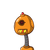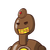# If the perimeter of a square is 28cm, then its side is i) 102cm iii) 7cm ii) 4cm iv) 14cm​

If the perimeter of a square is 28cm, then its side is i) 102cm iii) 7cm ii) 4cm iv) 14cm​

### 2 thoughts on “If the perimeter of a square is 28cm, then its side is i) 102cm iii) 7cm ii) 4cm iv) 14cm​”

1.Then side is (iii) 7 cm

2.7 cm

Step-by-step explanation:

perimeter of square (P)=28 cm

(P)= 4l

28=4l

l= 28/4

l= 7cm